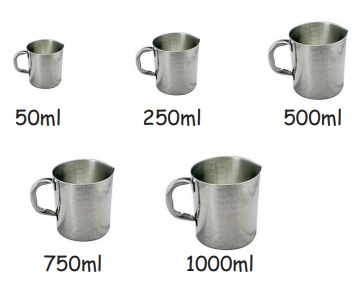Home | | Maths 5th Std | Capacity

# Capacity

Able to apply the four operation in solving problems involving capacity.

CAPACITY

Able to apply the four operation in solving problems involving capacity.

RecallLet us recall

10 millitire = 1 centilitre

10 centilitre =1 decilitre

10 decilitre = 1 litre

10 litre = 1 decalitre

10 decalitre = 1 hectalitre

10 hectalitre = 1 kilolitre

Let us know

1 litre = 1000ml

½ litre = 500ml

¼ litre = 250ml

¾ litre = 750ml

Tags : Measurements | Term 2 Chapter 4 | 5th Maths , 5th Maths : Term 2 Unit 4 : Measurements
Study Material, Lecturing Notes, Assignment, Reference, Wiki description explanation, brief detail
5th Maths : Term 2 Unit 4 : Measurements : Capacity | Measurements | Term 2 Chapter 4 | 5th Maths